By Matan Aspis | 8/3/2016 |

# Matplotlib Tutorial

Matplotlib Tutorial

Important Note: This tutorial has written at Spyder environment. (It's very comfortable, user friendly environment. therefore, it's highly recommended). As a result, there are some commands that occur automatically in this environment, and should be operated manually in other environments. I will emphasize some of them later on.

At First we would like to import the Matplotlib library in Ipython as follows:

Note that we also need to import the Numpy library for define arrays and vectors and the Math library to perform mathematical operations.

``````In : import numpy as np
In : import matplotlib.pyplot as plt
In : import math``````

We will draw a graph of the cos function at Section (-2π, 2π )

``````In : math.pi
Out: 3.141592653589793

In : x = np.linspace(-2*math.pi , 2*math.pi, 100)

In : y = np.cos(x)

In : plt.plot(x, y)
Out: [<matplotlib.lines.Line2D at 0x18e78048>]
``````

Notice thet the graphs display occurs inside the shell only because we work with the Spyder environment. In other environments we should use the magic function %matplotlib inline to do so. And in function plt.show() for creating the plot window which open automatically at spyder.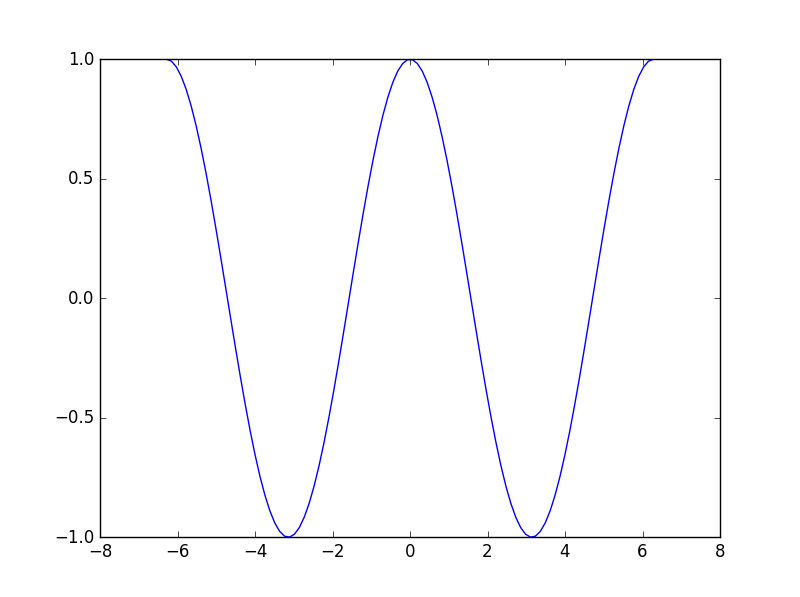Now we want to perform some operations on graphs as follows:

First lets reopen the plot window by the command:

``````In : plt.plot(x, y)
Out: [<matplotlib.lines.Line2D at 0xb530f28>]``````

Now lets perform several actions on the plot:

lets use the following magic command. It will open a separate window of the plot. Now we can activate the plot functions easily and effectively.

``````In : %matplotlib
Using matplotlib backend: Qt4Agg``````

All the actions will take place while the plot window is still open. after each command the plot will be updated accordingly

``````In : plt.title('Cosine plot',fontsize=18, color='y')
Out: <matplotlib.text.Text at 0x1b4cc588>

In : plt.ylabel('Y label',fontsize=12, color='g')
Out: <matplotlib.text.Text at 0x1b428588>

In : plt.xlabel('X label',fontsize=12, color='r')
Out: <matplotlib.text.Text at 0x1b212898>``````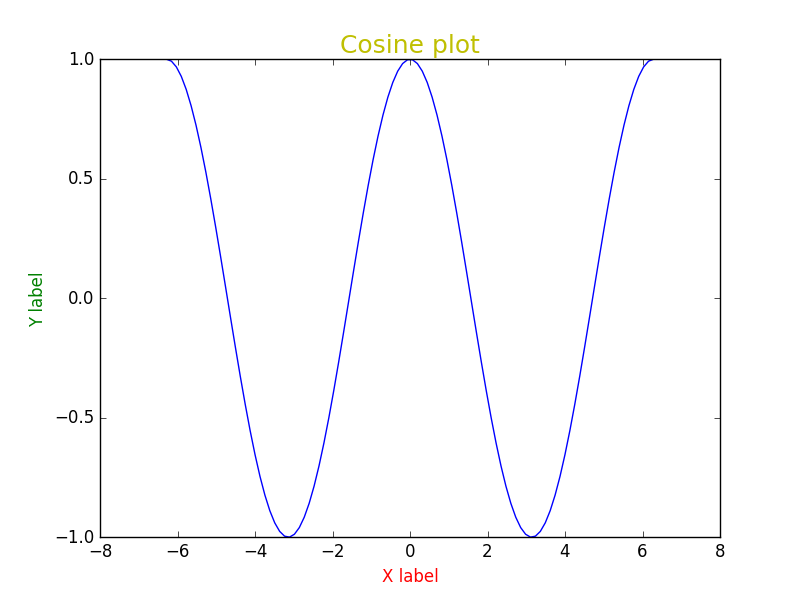``In : plt.colors?``
`````` =====   =======
Alias   Color
=====   =======
'b'     blue
'g'     green
'r'     red
'c'     cyan
'm'     magenta
'y'     yellow
'k'     black
'w'     white
=====   =======``````

Now we will want to get the plots of more than one function on the same chart:

``````In :x = np.linspace(0, 10, 11)

In :y = np.linspace(0, 10, 11)

In :plt.plot(x, y)
plt.plot(2*x, y)

Out: [<matplotlib.lines.Line2D at 0xc099d68>]``````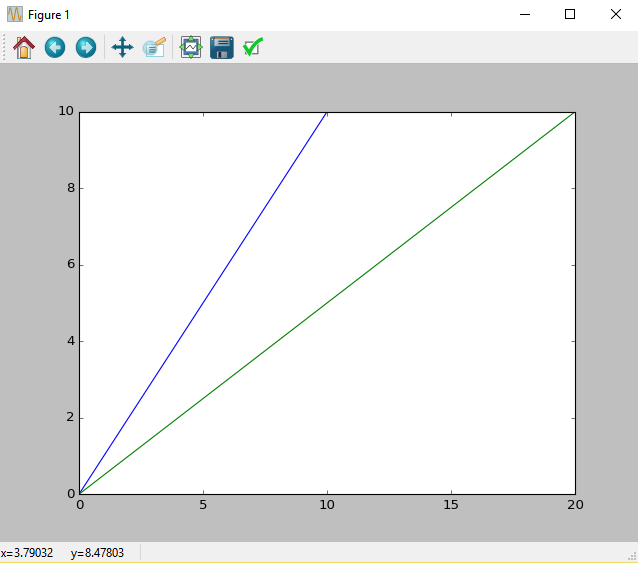We can also display the plots in two separate windows with the function plt.figure():

``````In :x = np.linspace(0, 10, 11)

In :y = np.linspace(0, 10, 11)

In :plt.plot(x, y)
plt.figure()
plt.plot(2*x, y)

Out: [<matplotlib.lines.Line2D at 0xccedbe0>]``````

We can create others marking graphs as follows:

``In : plt.plot(x, y, 'r^-')``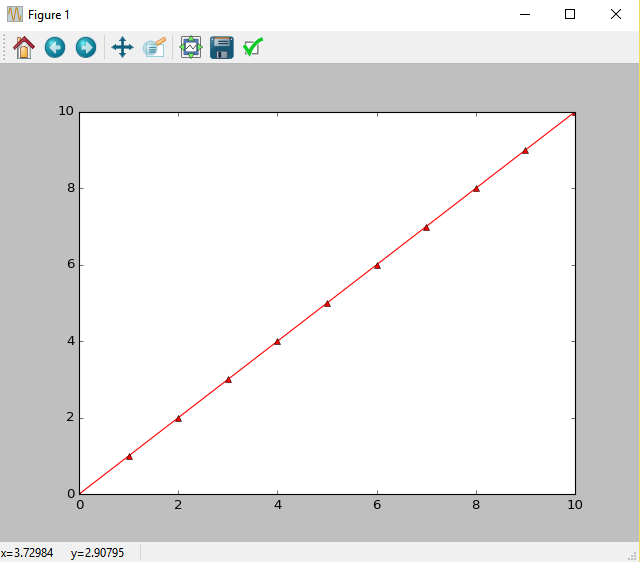The letter "r" describes the color curve. The Sign "^" describes the marking. Pay attention to what happens when skip the marking "-".:

Note that the order of the marks in the string does not matter.

``In : plt.plot(x, y, '^r')``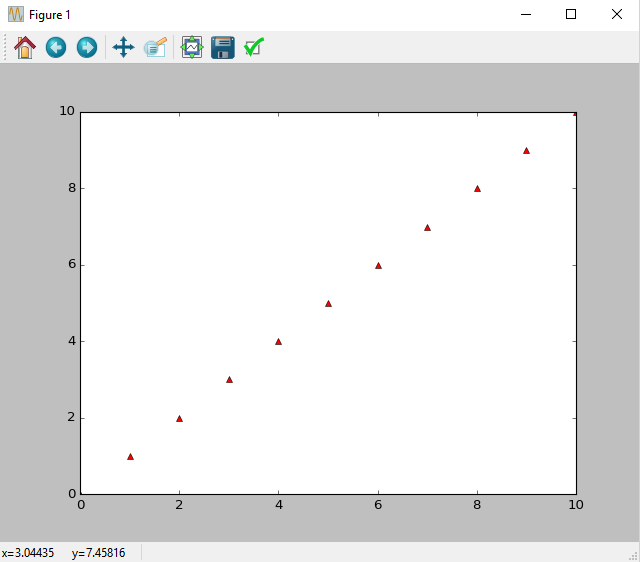Now we'll create a number of curves at the same graph with simple command:

We used " 'k-.' "  for displaying the curve with the following shap: "_ . _"

``In : plt.plot(x, np.sin(y), 'g^--', x, y, 'r*-', x,np.cos(y), 'k-.')``You can also design the marks themselves by the following shortcuts:

mec - the color of the marker perimeter
mfc - fill color of the marker
lw - line thickness of the curve
ms - The size of the marker
mew - the perimeter thickness of the of the marker

For additional design options, type the following command:

``````In : plt.plot?
``````

For example:

``````In : plt.plot(x**2, y, 'yo--',mec='green', mfc='red', mew=1,lw=2,ms=7)
Out: [<matplotlib.lines.Line2D at 0xca5e128>]``````Setting a Legend:

``````In : plt.plot(x, x**2, 'g^--', x, 8*np.sin(x), 'ro')
plt.legend(['x^2', '8*sin(x)'], loc='upper right')``````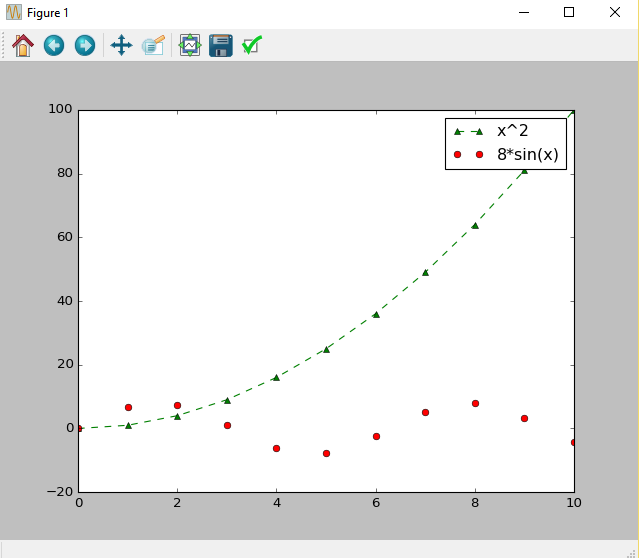The simplest way to define some curves in one graph.( the colors selected arbitrarily):

``````In : plt.plot(x, x*3, x, x*2, x, 5*x)
plt.legend(['5*x', '3*x','2*x'])``````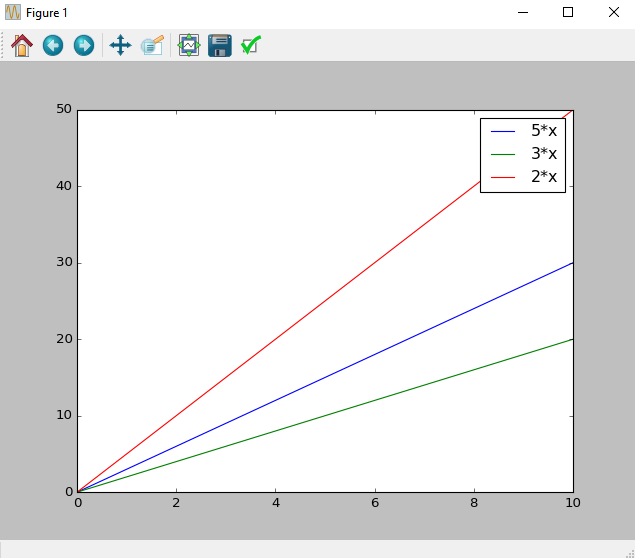Adding grid Lines to the graph:

``````In :plt.plot(x, x*3, x, x*2, x, 5*x)
plt.legend(['5*x', '3*x','2*x'])
plt.grid()``````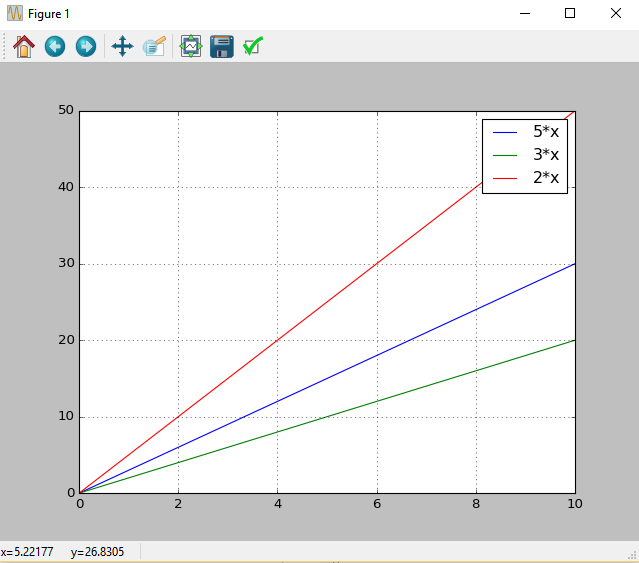## Recent Stories

### Top DiscoverSDK Experts3355
Ashton Torrence
Web and Windows developer
GUI | Web and 11 more
View Profile3220
Mendy Bennett
Mobile | Ad Networks and 1 more
View Profile3060
Karen Fitzgerald
7 years in Cross-Platform development.
Mobile | Cross Platform Frameworks
View Profile
X

## Compare Products

Select up to three two products to compare by clicking on the compare icon () of each product.

{{compareToolModel.Error}}

### Now comparing:

{{product.ProductName | createSubstring:25}} X
Compare Now